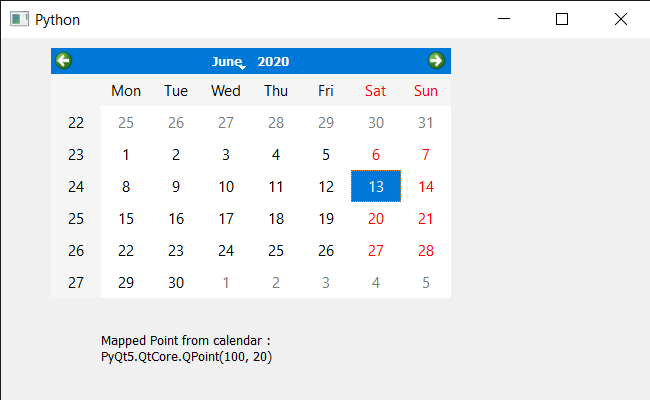# PyQt5 QCalendarWidget – Mapping co-ordinate system from Calendar co-ordinate system

• Last Updated : 17 Jun, 2020

In this article we will see how we can map the co-ordinate system from the QCalendarWidget. In order to do this we use `mapTo` method, this translates the widget coordinate position to the coordinate system of parent. The parent must not be None and must be a parent of the calling widget.

In order to do this we will use `mapTo` method with the QCalendarWidget object.

Attention geek! Strengthen your foundations with the Python Programming Foundation Course and learn the basics.

To begin with, your interview preparations Enhance your Data Structures concepts with the Python DS Course. And to begin with your Machine Learning Journey, join the Machine Learning - Basic Level Course

Syntax : calendar.mapTo(parent, point)

Argument : It takes QWidget object that is parent and the QPoint object as argument

Return : It return QPoint object

Below is the implementation

 `# importing libraries``from` `PyQt5.QtWidgets ``import` `*` `from` `PyQt5 ``import` `QtCore, QtGui``from` `PyQt5.QtGui ``import` `*` `from` `PyQt5.QtCore ``import` `*` `import` `sys`` ` `# QCalendarWidget Class``class` `Calendar(QCalendarWidget):`` ` `    ``# constructor``    ``def` `__init__(``self``, parent ``=` `None``):``        ``super``(Calendar, ``self``).__init__(parent)`` ` ` ` `class` `Window(QMainWindow):`` ` `    ``def` `__init__(``self``):``        ``super``().__init__()`` ` `        ``# setting title``        ``self``.setWindowTitle(``"Python "``)`` ` `        ``# setting geometry``        ``self``.setGeometry(``100``, ``100``, ``650``, ``400``)`` ` `        ``# calling method``        ``self``.UiComponents()`` ` `        ``# showing all the widgets``        ``self``.show()`` ` `    ``# method for components``    ``def` `UiComponents(``self``):`` ` `        ``# creating a QCalendarWidget object``        ``# as Calendar class inherits QCalendarWidget``        ``self``.calendar ``=` `Calendar(``self``)`` ` `        ``# setting geometry to the calender``        ``self``.calendar.setGeometry(``50``, ``10``, ``400``, ``250``)`` ` `        ``# setting cursor``        ``self``.calendar.setCursor(Qt.PointingHandCursor)`` ` `        ``# creating label to show the properties``        ``self``.label ``=` `QLabel(``self``)`` ` `        ``# setting geometry to the label``        ``self``.label.setGeometry(``100``, ``280``, ``250``, ``60``)`` ` `        ``# making label multi line``        ``self``.label.setWordWrap(``True``)`` ` `        ``# mapping from calendar co-ordinate system``        ``value ``=` `self``.calendar.mapTo(``self``, QPoint(``50``, ``10``))`` ` `        ``# setting text to the label``        ``self``.label.setText(``"Mapped Point from calendar : "` `+` `str``(value))`` ` ` ` ` ` `# create pyqt5 app``App ``=` `QApplication(sys.argv)`` ` `# create the instance of our Window``window ``=` `Window()`` ` `# start the app``sys.exit(App.``exec``())`

Output :My Personal Notes arrow_drop_up